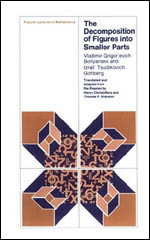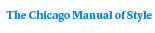# The Decomposition of Figures Into Smaller Parts80 pages | 6 x 9 | © 1980
Paper \$28.00 ISBN: 9780226063577 Published April 1980
In contrast to the vast literature on Euclidean geometry as a whole, little has been published on the relatively recent developments in the field of combinatorial geometry. Boltyanskii and Gohberg’s book investigates this area, which has undergone particularly rapid growth in the last thirty years. By restricting themselves to two dimensions, the authors make the book uniquely accessible to interested high school students while maintaining a high level of rigor. They discuss a variety of problems on figures of constant width, convex figures, coverings, and illumination. The book offers a thorough exposition of the problem of cutting figures into smaller pieces. The central theorem gives the minimum number of pieces into which a figure can be divided so that all the pieces are of smaller diameter than the original figure. This theorem, which serves as a basis for the rest of the material, is proved for both the Euclidean plane and Minkowski’s plane.
Table of Contents• Contents
Contents
Preface
1. Division of Figures into Pieces of Smaller Diameter
1.1. The Diameter of a Figure
1.2. Formulation of the Problem
1.3. Borsuk’s Theorem
1.4. Convex Figures
1.5. Figures of Constant Width
1.6. Embedding in a Figure of Constant Width
1.7. For Which Figures is a(F) = 3?
2. Division of Figures in the Minkowski Plane
2.1. A Graphic Example
2.2. The Minkowski Plane
2.3. Borsuk’s Problem in Minkowski Planes
3. The Covering of Convex Figures by Reduced Copies
3.1. Formulation of the Problem
3.2. Another Formulation of the Problem
3.3. Solution of the Covering Problem
3.4. Proof of Theorem 2.2
4. The Problem of Illumination
4.1. Formulation of the Problem
4.2. Solution of the Problem of Illumination
4.3. The Equivalence of the Last Two Problems
4.4. Division and Illumination of Unbounded Convex Figures
Remarks